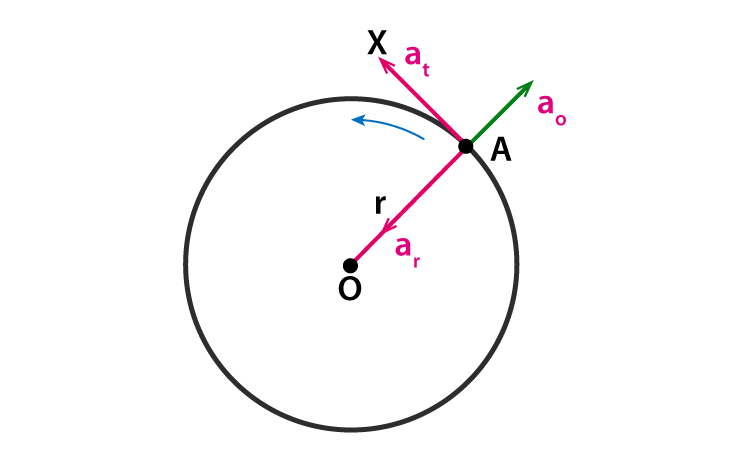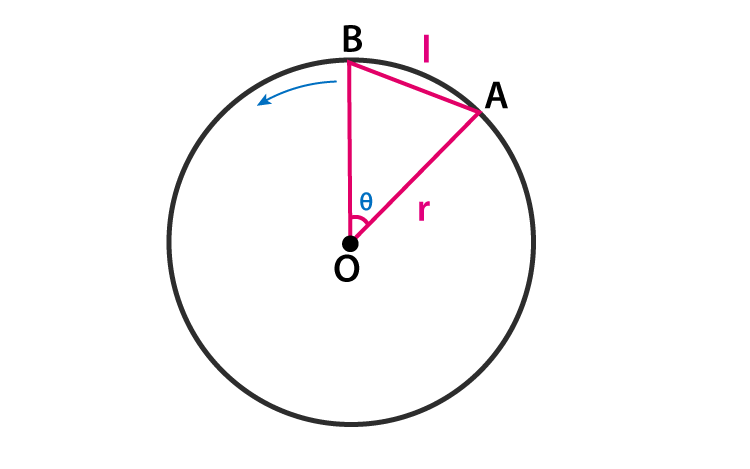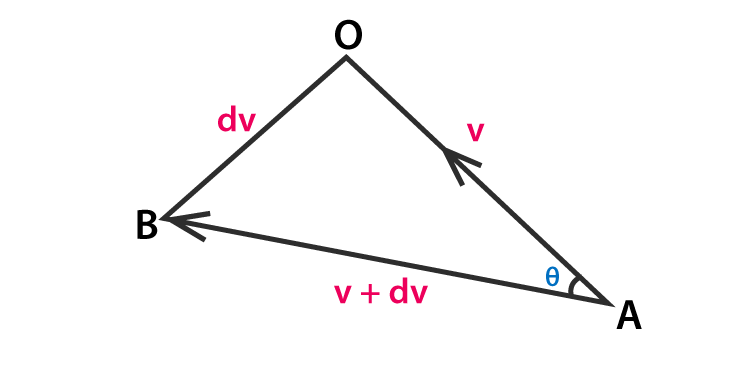# Radial Acceleration - Formula, Derivation, Units

The object under motion can undergo a change in its speed. The measure of the rate of change in its speed along with direction with respect to time is called acceleration. The motion of the object can be linear or circular. Thus, the acceleration involved in linear motion is called linear acceleration. The acceleration involved in a circular motion is called angular acceleration.

## Introduction

If we recall circular motion, here distance covered will be along the curved path (circumference of a circle). The velocity involved is angular velocity and not linear velocity. Similarly, the acceleration involved is centripetal acceleration. Some important terms involved are-

Terms Definition Symbol Formula Units

### Angular displacement

The angular difference between the initial and final position of an object. 𝜃
$$\begin{array}{l}\theta =\frac{s}{r}\end{array}$$

### Angular velocity

The rate of change of angular displacement with respect to time.
$$\begin{array}{l}\omega =\frac{d\theta }{dt}\end{array}$$

### Angular acceleration

The rate of change of angular velocity with respect to time. 𝛼
$$\begin{array}{l}\alpha =\frac{d\omega }{dt}\end{array}$$

In a circular motion, the acceleration experienced by the body towards the centre is called the centripetal acceleration. This can be resolved into two-component. A radial component and a tangential component depend upon the type of motion.

In a uniform circular motion, “the acceleration of the object is along the radius, directed towards the centre” is called radial acceleration.figure(1) schematic diagram representing an object under rotational motion

An object A is tied to a string and made to rotate about a fixed point O (centre) (see figure(1)). The object when rotated fast, the string (OA) looks almost like the radius of the circle, which implies that a force is exerted on the object from the centre, thereby an acceleration a0 along the radial direction (along the radius of the circle towards the centre). To counter this force, tension is developed along the string in the opposite direction. This force due to tension is called centripetal force, thus the acceleration generated on the object is called centripetal acceleration or radial acceleration ar.figure(2) Positions of two objects A and B. They are infinitesimally close.figure(3) The equivalent diagram of the centripetal velocity vector A and B.

Implementing the property of similar triangles we get-

$$\begin{array}{l}\frac{AB}{OA}=\frac{l}{r}\end{array}$$

Since A and B are infinitesimally close, we can approximate AB to the length of arc AB.

$$\begin{array}{l}AB=v\times dt\end{array}$$

From figure(3) since A and B are very close,

$$\begin{array}{l}v+dv\approx dv\end{array}$$
$$\begin{array}{l}\frac{AB}{OA}=\frac{dv}{v}\end{array}$$
$$\begin{array}{l}\frac{v\times dt}{r}=\frac{dv}{v}\end{array}$$

On rearranging,

$$\begin{array}{l}\frac{dv}{dt}=\frac{v^{2}}{r}\end{array}$$

Thus,

$$\begin{array}{l}\frac{dv}{dt}\end{array}$$
gives radial acceleration of an object under uniform circular motion. We arrive at the final expression-

 $$\begin{array}{l}a_{r}=\frac{v^{2}}{r}\end{array}$$

## Tangential Component

The tangential component is defined as the component of angular acceleration tangential to the circular path. The unit of measurement is m.s-2. The mathematical representation is given as:

$$\begin{array}{l}a_{t}=\frac{v_{2}-v_{1}}{t}\end{array}$$
Where,

• at is the tangential component
• t is the time period
• v1 and v2 are the respective velocities of the two objects in a circular motion

Radial Acceleration has the unit same as that of angular acceleration is radian/sec2

Physics Related Topics:

## Understand uniform circular motion with the help of real-life examples.## Frequently Asked Questions – FAQs

### What is acceleration?

Acceleration is defined as the rate of change of motion of a body. In other words, the measure of the rate of change in its speed along with direction with respect to time is called acceleration.

### What are the main types of acceleration?

Linear acceleration and circular acceleration are the main types of acceleration.

### What is linear acceleration?

The motion of the object can be linear or circular. Thus, the acceleration involved in linear motion is called linear acceleration.

### What is circular acceleration?

The acceleration involved in a circular motion is called angular acceleration.